Download Presentation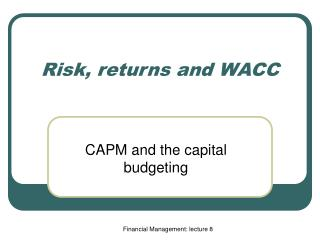Risk, returns and WACC

# Risk, returns and WACC - PowerPoint PPT Presentation

Risk, returns and WACC. CAPM and the capital budgeting. Today’s plan. Review what we have learned in the last lecture CAPM and the expected return The security market line The application of CAPM in capital budgeting WACC. What have we learned in the last lecture?.I am the owner, or an agent authorized to act on behalf of the owner, of the copyrighted work described.
Download Presentation## Risk, returns and WACC

An Image/Link below is provided (as is) to download presentation

Download Policy: Content on the Website is provided to you AS IS for your information and personal use and may not be sold / licensed / shared on other websites without getting consent from its author.While downloading, if for some reason you are not able to download a presentation, the publisher may have deleted the file from their server.

- - - - - - - - - - - - - - - - - - - - - - - - - - E N D - - - - - - - - - - - - - - - - - - - - - - - - - -
Presentation Transcript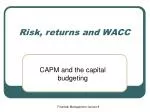### Risk, returns and WACC

CAPM and the capital budgeting

Financial Management: lecture 8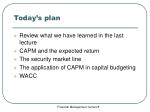Today’s plan
• Review what we have learned in the last lecture
• CAPM and the expected return
• The security market line
• The application of CAPM in capital budgeting
• WACC

Financial Management: lecture 8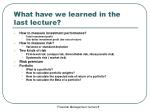What have we learned in the last lecture?
• How to measure investment performance?
• Total investment profit
• One dollar investment profit (the rate of return)
• How to measure risk?
• Variance or standard deviation
• Two kinds of risk
• Firm-level risk (unique risk, diversifiable risk, idiosyncratic risk)
• Systematic risk ( market risk)
• Portfolio
• What is a portfolio?
• How to calculate portfolio weights?
• How to calculate the expected rate of return of a portfolio?
• How to calculate the Beta of a portfolio?

Financial Management: lecture 8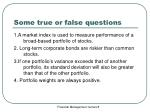Some true or false questions

1.A market index is used to measure performance of a broad-based portfolio of stocks.

2. Long-term corporate bonds are riskier than common stocks.

3.If one portfolio's variance exceeds that of another portfolio, its standard deviation will also be greater than that of the other portfolio.

4. Portfolio weights are always positive.

Financial Management: lecture 8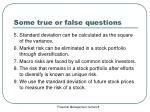Some true or false questions

5. Standard deviation can be calculated as the square of the variance.

6. Market risk can be eliminated in a stock portfolio through diversification.

7. Macro risks are faced by all common stock investors.

8. The risk that remains in a stock portfolio after efforts to diversify is known as unique risk.

9. We use the standard deviation of future stock prices to measure the risk of a stock.

Financial Management: lecture 8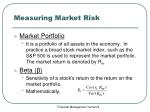Measuring Market Risk
• Market Portfolio
• It is a portfolio of all assets in the economy. In practice a broad stock market index, such as the S&P 500 is used to represent the market portfolio. The market return is denoted by Rm
• Beta (β)
• Sensitivity of a stock’s return to the return on the market portfolio,
• Mathematically,

Financial Management: lecture 8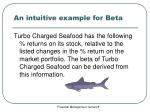An intuitive example for Beta

Turbo Charged Seafood has the following % returns on its stock, relative to the listed changes in the % return on the market portfolio. The beta of Turbo Charged Seafood can be derived from this information.

Financial Management: lecture 8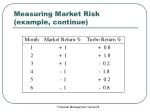Measuring Market Risk (example, continue)

Financial Management: lecture 8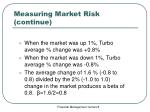Measuring Market Risk (continue)
• When the market was up 1%, Turbo average % change was +0.8%
• When the market was down 1%, Turbo average % change was -0.8%
• The average change of 1.6 % (-0.8 to 0.8) divided by the 2% (-1.0 to 1.0) change in the market produces a beta of 0.8. β=1.6/2=0.8

Financial Management: lecture 8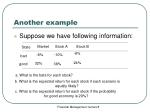Another example
• Suppose we have following information:

Market

Stock A

Stock B

State

-6%

-8%

-10%

24%

32%

good

38%

a. What is the beta for each stock?

b. What is the expected return for each stock if each scenario is

equally likely?

c. What is the expected return for each stock if the probability

for good economy is 20%?

Financial Management: lecture 8Solution

a.

b.

c.

Financial Management: lecture 8Portfolio Betas
• Diversification reduces unique risk, but not market risk.
• The beta of a portfolio will be an weighted average of the betas of the securities in the portfolio.
• What is the beta of the market portfolio?
• What is the beta of the risk-free security?

Financial Management: lecture 8Example
• Suppose you have a portfolio of IBM and Dell with a beta of 1.2 and 2.2, respectively. If you put 50% of your money in IBM, and the other in Dell, what is the beta of your portfolio

Beta of your portfolio =0.5*1.2 +0.5*2.2=1.7

Financial Management: lecture 8Market risk and risk premium
• Risk premium for bearing market risk
• The difference between the expected return required by investors and the risk-free asset.
• Example, the expected return on IBM is 10%, the risk-free rate is 5%, and the risk premium is 10% -5%=5%
• If a security ( an individual security or a portfolio) has market or systematic risk, risk-averse investors will require a risk premium.

Financial Management: lecture 8CAPM (Capital Asset Pricing Model)
• The risk premium on each security is proportional to the market risk premium and the beta of the security.
• That is,

Financial Management: lecture 8Security market line
• The graphic representation of CAPM in the expected return and Beta plane

Security Market Line

Rm

rf

Financial Management: lecture 8Project Risk and cost of the capital
• In capital budgeting, in order to calculate the NPV of the project, we need to measure the risk of the project and thus find out the discount rate (the cost of capital)
• We can use Beta of the project cash flows to measure the risk of the project and use CAPM to get the expected return required by investors

Financial Management: lecture 8Example 1
• Based on the CAPM, ABC Company has a cost of capital of 17%. (4 + 1.3(10)). A breakdown of the company’s investment projects is listed below.
• 1/3 Nuclear Parts: β=2.0
• 1/3 Computer Hard Drive: β =1.3
• 1/3 Dog Food Production: β =0.6
• When evaluating a new dog food production investment, which cost of capital should be used and how much?

Financial Management: lecture 8Solution
• Since dog food projects may have similar systematic risk to the dog food division, we use a beta of 0.6 to measure the risk of the projects to be taken.
• Thus the expected return on the project or the cost of capital is 0.04+0.6*(0.1)=0.l or 10%

Financial Management: lecture 8Example 2
• Stock A has a beta of .5 and investors expect it to return 5%. Stock B has a beta of 1.5 and investors expect it to return 13%. What is the market risk premium and the expected rate of return on the market portfolio?

Financial Management: lecture 8Solution
• According to the CAPM

Financial Management: lecture 8Example 3
• You have \$1 million of your own money and borrow another \$1 million at a risk-free rate of 4% to invest in the market portfolio. The expected return for the market portfolio is 12%, what is the expected return on your portfolio?

Financial Management: lecture 8Solution
• We can use two approaches to solve it:
• First, the expected rate of return of a portfolio is the weighed average of the expected rates of return of the securities in the portfolio.
• Second , the beta of a portfolio is the weighed average of the betas of the securities in the portfolio. Then use the CAPM to get the expected rate of return.

Financial Management: lecture 8Solution (continue)
• First approach
• Second approach

Financial Management: lecture 8The cost of capital

Cost of Capital

• The expected return the firm’s investors require if they invest in securities or projects with comparable degrees of risk.

Financial Management: lecture 8The cost of capital for the bond
• The cost of capital for the bond
• It is the YTM, the expected return required by the investors.
• That is
• The expected return on a bond can also be calculated by using CAPM

Financial Management: lecture 8Example 2
• A bond with a face value of \$2000 matures in 5 years. The coupon rate is 8%. If the market price for this bond is \$1900.

(a) What is the expected return on this bond or what is the cost of debt or interest rate for this bond?

(b) Suppose that the YTM is 9%, what is the market value of this bond?

Financial Management: lecture 8Solution

(a)

(b)

Financial Management: lecture 8The cost of capital for a stock
• The cost of capital for a stock is calculated by using
• CAPM
• Dividend growth model

Financial Management: lecture 8Example 3
• Sock A now pays a dividend of \$1.5 per share annually, It is expected that dividend is going to grow at a constant rate of 2%. The current price for stock A is \$25 per share. What is the expected return or the cost of capital by investing in this stock?

Financial Management: lecture 8Solution

Using the dividend discount model, we have

Financial Management: lecture 8Capital structure
• Capital Structure
• The firm’s mix of debt financing and equity financing.
• If we use D , E and V to denote the values of debt, equity and firm (asset), respectively.
• Then V=D+E
• The ratio of D/V or D/E can be used to measure the capital structure of the firm.

Financial Management: lecture 8Weighted Average Cost of Capital (WACC)
• If you invest in a stock, you require an expected rate of return, or the cost of capital for your investment in the stock
• If you invest in a bond, you require an expected rate of return, or the cost of capital for the bond
• Then, if you invest in a portfolio of all the firm’s securities (debt and stock), what is the expected rate of return you require?
• The expected rate of return on a portfolio of all the firm’s securities is called the weighted average cost of capital

(WACC). It is also called the expected return on the asset of the firm.

Financial Management: lecture 8WACC (continues)
• According to portfolio theory, we know the expected return on a portfolio is the weighted average of the expected returns on the securities of the portfolio.
• Thus, WACC for a firm with debt and equity is

Financial Management: lecture 8WACC (continue)
• In calculating WACC, we have to use market values of debt and equity.
• Even if you are given the book value of debt, you may convert this book value to market debt value to calculate WACC
• Why do we use market values of debt and equity, but not book values of debt and equity, in calculating WACC?

Financial Management: lecture 8Example 4
• Geothermal Inc. has two securities: debt and stocks. The market debt value is \$194 million, but the firm’s market value is \$647 million. Given that geothermal pays 8% for debt and 14% for equity, what is the Company Cost of Capital (There is no corporate tax)?

Financial Management: lecture 8Solution

Financial Management: lecture 8Example 5
• Executive Fruit has issued debt, preferred stock and common stock. The market value of these securities are \$4mil, \$2mil, and \$6mil, respectively. The required returns are 6%, 12%, and 18%, respectively.
• What is the WACC for Executive Fruit, Inc.?

Financial Management: lecture 8Solution

Financial Management: lecture 8Summary of WACC calculation
• Three steps in calculating WACC
• First step: Calculate the portfolio weight using the market value.
• Second step: Determine the required rate of return on each security in the portfolio.
• Third step: Calculate a weighted average of these returns, or the expected return on the portfolio.

Financial Management: lecture 8The impact of tax on WACC
• Taxes are an important consideration in the company cost of capital because interest payments are deducted from income before tax is calculated.

Financial Management: lecture 8WACC with tax

Weighted -average cost of capital=

Financial Management: lecture 8Example 6 (with tax)
• Geothermal Inc. has two securities: debt and stocks. The market debt value is \$194 million, but the firm’s market value is \$647 million. Given that geothermal pays 8% for debt and 14% for equity, what is the Company Cost of Capital if the tax rate is 50%?

Financial Management: lecture 8Solution

Financial Management: lecture 8